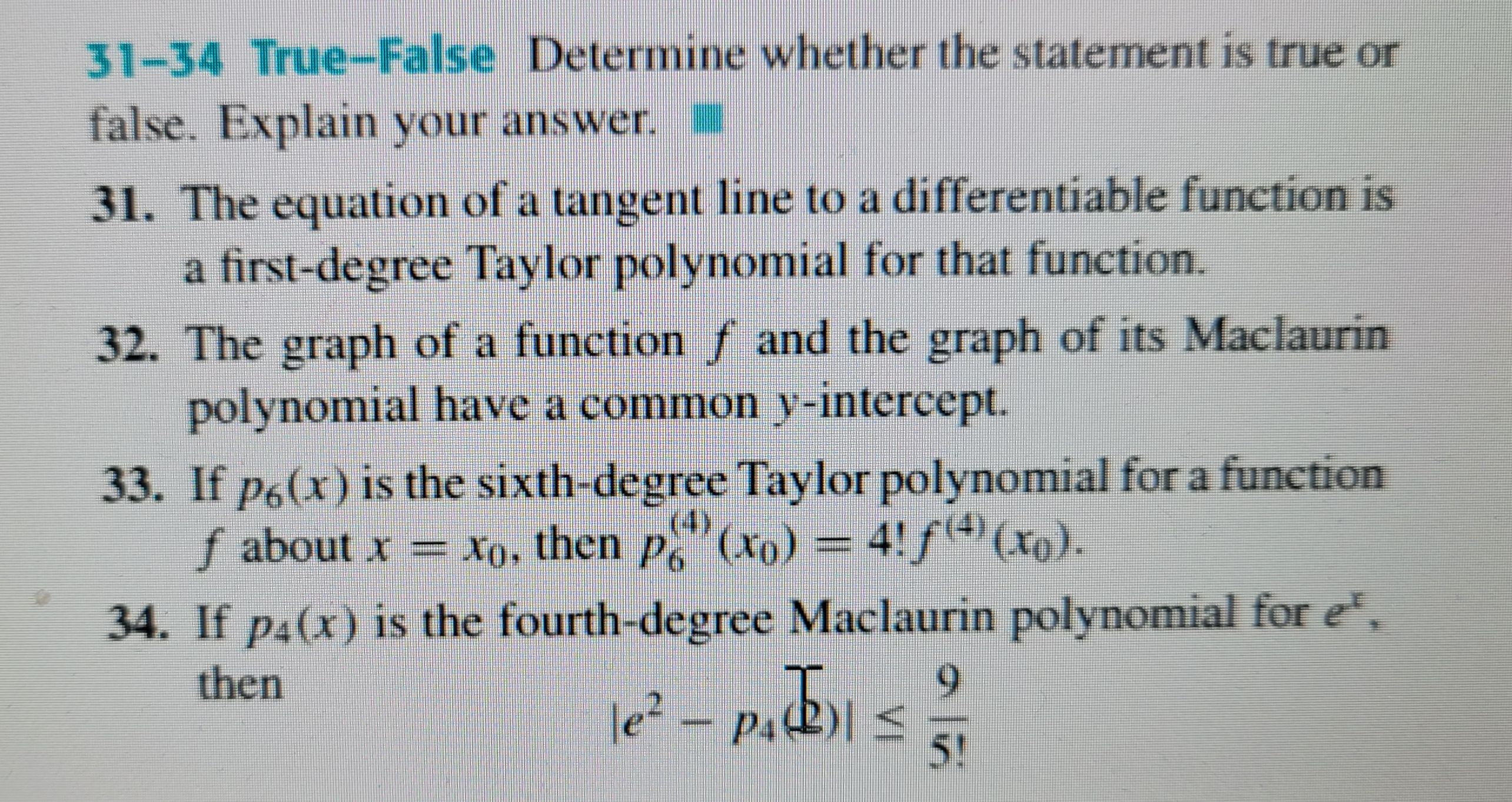### ¿Todavía tienes preguntas de matemáticas?

Pregunte a nuestros tutores expertos
Algebra
Pregunta31. The equation of a tangent line to a differentiable function is a first-degree Taylor polynomial for that function.

$$f(x)\approx g(x)= f(x_{0})+ f'(x_{0})(x- x_{0})\\ g(x_{0})= f(x_{0})\\g'(x_{0})= f'(x_{0)}$$